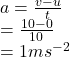## david starts at rest and increase it’s speed to 10 m/s in 10s. what is it’s acceleration?​

Question

david starts at rest and increase it’s speed to 10 m/s in 10s. what is it’s acceleration?​

in progress 0
2 weeks 2021-08-29T19:08:49+00:00 2 Answers 1 views 0

1. Hey!!

Solution,

Initial velocity(u)=0 m/s

Final velocity(v)=10m/s

Time(t)=10s

Acceleration=?

Now,

Acceleration=v-u/t

=100/10

= 10/10

=1 m/s^2

The acceleration is 1 m/s^2

Hope it helps